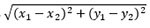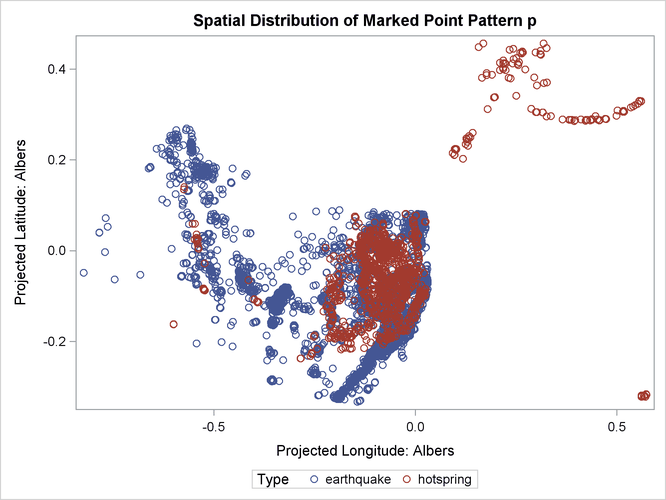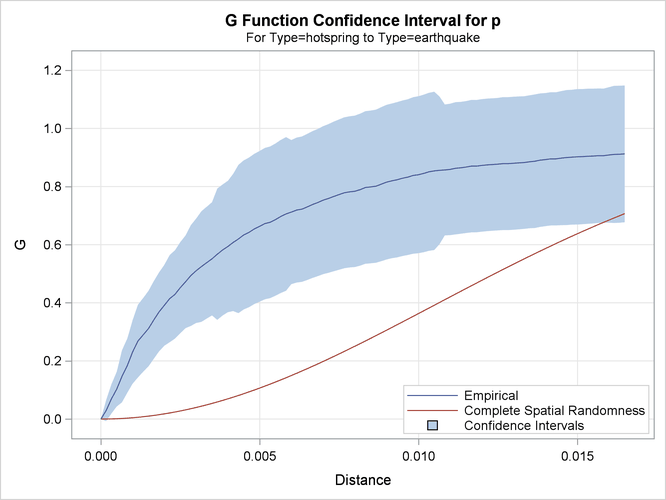SAS/STAT Examples

Handling Spatial Data in Spherical Coordinates

Contents | SAS Program | PDF

Overview

PROC SPP and other spatial analysis procedures in SAS/STAT are designed to handle projected coordinate systems, where the distance between two points can be computed using the Euclidean formula,. If your data are collected in a spherical coordinate system—for example, longitude and latitude—then you should convert it to a projected system before applying PROC SPP. This example walks you through a sequence of steps that demonstrate how to handle data that have spherical coordinates in order to analyze them by using PROC SPP.

Example

You are a geologist studying the relationship between the locations of earthquakes and the locations of geothermal activity in the western United States. You have earthquake data, courtesy of the United States Geological Service (USGS) and data about hot springs, courtesy of the National Oceanic and Atmospheric Administration (NOAA).

The Earthquakes data set is the collection of earthquakes with magnitude greater than 2.5 (on the Richter scale) in the continental United States collected from 2005 to 2015. In addition to the latitude and longitude of the epicenter of each earthquake, the data include other attributes, such as the earthquake’s magnitude. The following DATA step reads the data and creates the data set Earthquakes:


data earthquakes;
length Type $10; infile "https://support.sas.com/rnd/app/data/earthquakes.txt" url; input Latitude Longitude Depth Magnitude dNearestStation RootMeanSquareTime Type$;
run;


The Hotsprings data set is the collection of hot spring locations in the continental United States. Again, along with the latitude and longitude of each hot spring, the data include other attributes, such as its temperature and popular name. The following DATA step reads the data and creates the data set Hotsprings:


data hotsprings;
length Type $10; infile "https://support.sas.com/rnd/app/data/hotsprings.txt" url; input Latitude 1-6 Longitude 8-15 TemperatureFarenheit$ 17-19
TemperatureCelsius \$ 21-23;
Type = "hotspring";
run;


You can view both of the data sets as spatial point patterns that are given in spherical coordinates. To explore whether the locations of hot springs and earthquakes are correlated, you first merge the two data sets into a single marked spatial point pattern, with a type variable to denote an earthquake, explosion, landslide, or hot spring, as shown in the following code.


data QuakesAndSprings;
set earthquakes hotsprings;
where (type in ('hotspring','earthquake'));
run;


The combined data set, QuakesAndSprings, contains locations in spherical coordinates, given as Longitude and Latitude. You can use the GPROJECT procedure in SAS/GRAPH® to transform these spherical coordinates to projected coordinates. PROC GPROJECT requires that the data have an identification variable and that the spherical coordinates be named X and Y, respectively. The following statements prepare the data, apply PROC GPROJECT, and then prepare the resulting projected data for analysis by PROC SPP:


data GProjectIn;
set QuakesAndSprings;
ID = _N_;
rename latitude=y longitude=x;
run;

proc gproject data=GProjectIn out=GProjectOut degrees;
id ID;
run;

data GProjectOut;
set GProjectOut;
format _character_;
informat _character_;
run;


The resulting data set, GProjectOut, contains the data in projected coordinates. In this form, you can use the projected data with PROC SPP to analyze the relationship between earthquakes and hot springs by using the following statements


ods graphics on;
proc spp data=GProjectOut plots(unpack)=(all observ(attr=mark));
process p = (X,Y / mark=type)
/ G cross=types('hotspring','earthquake') maxdist=max;
run;


Figure 1: Projected locations of earthquakes and hot springsFigure 1 shows the locations of earthquakes, hot springs, landslides and explosions of different kinds.

Figure 2: Cross G-function between Hot Spring and EarthquakeFigure 2 shows the plot of the edge-corrected cross G-function computed between earthquakes and hot springs. The blue line, which represents the empirical cross G-function, is far above the dashed red line. The confidence interval of the cross G-function is shown by the blue band around the blue line, also does not intersect with the dashed red line. This suggests that earthquakes are indeed clustered around hot spring locations.